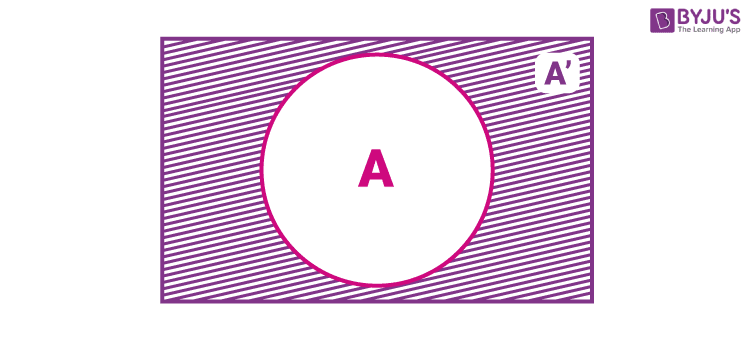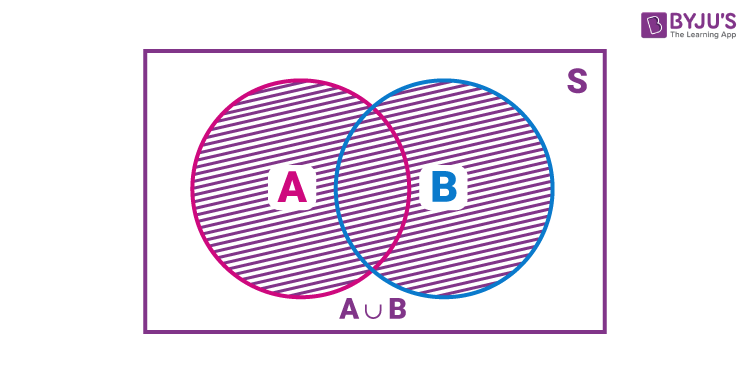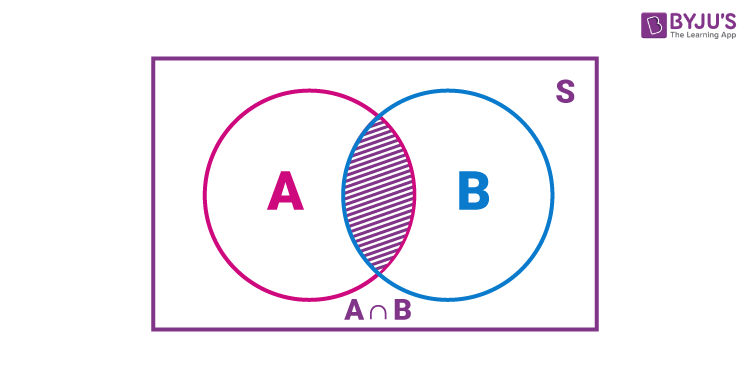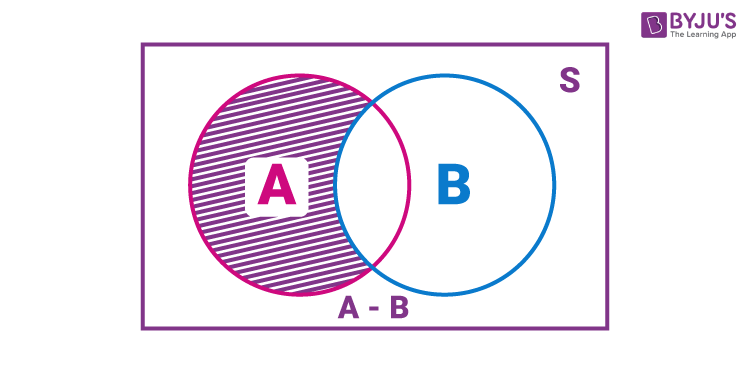# Algebra of Events

In earlier classes, we might have studied various techniques of combining two or more sets, viz, union, intersection, difference, the complement of a set, etc. Likewise we can connect two or more events by using similar set notations. Before learning about the algebra of events, recall the meaning of event in probability and how to represent it.

What is an event in Probability?

In probability, any subset, say E of a sample space S, is called an event. We have different types of events in probability. Also, we can perform different operations on these called the algebra of events.

## Algebra of Events in Probability

The algebra of events in probability comprises the following:

• Complementary Event
• The Event ‘A or B’
• The Event ‘A and B’
• The Event ‘A but not B’

Suppose A, B, and C are the events associated with an experiment whose sample space is S such that A ⊆ S, B ⊆ S and C ⊆ S that means subsets of S. Let’s define the algebra of these events one by one.

### Complementary Event

For every event A, there corresponds another event A′ called the complementary event to A. It is also called the event ‘not A’.

A′ = {ω : ω ∈ S and ω ∉A} = S – A

Here, A is the event, ω is the outcome associated with the event A and S is the sample space.

We can show the complementary event using venn diagram as:For example, consider the experiment of ‘tossing three coins’.

The associated sample space can be written as:

S = {HHH, HHT, HTH, THH, HTT, THT, TTH, TTT}

Let A be the event of getting ‘at least two heads’.

Thus, the outcomes associated with the event A = {HHT, HTH, THH, HHH}

From this, we can say that, for the outcome HTT the event A has not occurred. In other words, we may say that the event ‘not A’ has occurred.

Therefore, the complementary event ‘not A’ of the event A is A′.

i.e. A′ = {THH, HTT, THT, TTH, TTT}

### The Event ‘A or B’

The Event ‘A or B’ is the union of two sets A and B, denoted by A ∪ B containing all those elements in A or B or both.

When sets A and B are two events associated with a sample space, then A ∪ B is either A or B or both. This event ‘A ∪ B’ is also called ‘A or B’.

Therefore Event ‘A or B’ = A ∪ B = {ω: ω ∈ A or ω ∈ B}

The venn diagram representation of A or B, i.e. A ∪ B is given below:For example, consider the experiment of throwing two dice at a time.

The associated sample space can be written as:

S = {(1, 1), (1, 2), (1, 3), (1, 4), (1, 5), (1, 6), (2, 1), (2, 2), (2, 3), (2, 4), (2, 5), (2, 6), (3, 1), (3, 2), (3, 3), (3, 4), (3, 5), (3, 6), (4, 1), (4, 2), (4, 3), (4, 4), (4, 5), (4, 6), (5, 1), (5, 2), (5, 3), (5, 4), (5, 5), (5, 6), (6, 1), (6, 2), (6, 3), (6, 4), (6, 5), (6, 6)}

Let A be the event of getting a sum of two scores will be a multiple of 3 and B be the event of getting same scores on both dice.

The outcomes associated with these events are:

A = {(1, 2), (2, 1), (1, 5), (5, 1), (2, 4), (4, 2), (3, 3), (3, 6), (6, 3), (4, 5), (5, 4), (6, 6)}

B = {(1, 1), (2, 2), (3, 3), (4, 4), (5, 5), (6, 6)}

Therefore, A ⋃ B = {(1, 1), (1, 2), (1, 5), (2, 1), (2, 2), (2, 4), (3, 3), (3, 6), (4, 2), (4, 4), (4, 5), (5, 1), (5, 4), (5, 5), (6, 3), (6, 6)}

### The Event ‘A and B’

The Event ‘A and B’ is the intersection of two sets A ∩ B is the set of those common elements to both A and B, i.e., the elements belong to both ‘A and B’.

If A and B are two events, then the set A ∩ B denotes the event ‘A and B’. It is expressed as:

A ∩ B = {ω: ω ∈ A and ω ∈ B}

Below figure shows the set A ∩ B using Venn diagram.Again, consider the experiment of throwing two dice at a time.

The associated sample space can be written as:

S = {(1, 1), (1, 2), (1, 3), (1, 4), (1, 5), (1, 6), (2, 1), (2, 2), (2, 3), (2, 4), (2, 5), (2, 6), (3, 1), (3, 2), (3, 3), (3, 4), (3, 5), (3, 6), (4, 1), (4, 2), (4, 3), (4, 4), (4, 5), (4, 6), (5, 1), (5, 2), (5, 3), (5, 4), (5, 5), (5, 6), (6, 1), (6, 2), (6, 3), (6, 4), (6, 5), (6, 6)}

Let A be the event of getting a score on the second die is 5 and B be the event of getting sum of scores on dice is 10 & more than 10.

The outcomes associated with these events are:

A = {(1, 5), (2, 5), (3, 5), (4, 5), (5, 5), (6, 5)}

B = {(3, 6), (6, 4), (5, 5), (5, 6), (6, 5), (6, 6)}

Therefore, A ∩ B = {(5, 5), (6, 5)}

### The Event ‘A but not B’

The event A but not B is the set A – B, i.e. difference of sets A and B. It is the set of all those elements which are in A but not in B. We can calculate the set A – B using the expression:

A – B = A ∩ B′

Thus, A – B = {ω: ω ∈ A and ω ∉ B}

The below figure helps in understanding the event A but not B.Let us consider the experiment of rolling a die.

The sample space is S = {1, 2, 3, 4, 5, 6}

Let A be the event of getting a prime number and B be the event of getting an even number.

The outcomes associated with these events are:

A = {2, 3, 5}

B = {2, 4, 6}

Thus, A – B = {2, 3, 5} – {2, 4, 6} = {3, 5}

Alternatively,

B′ = {1, 3, 5}

A ∩ B′ = { 2, 3, 5} ∩ {1, 3, 5} = {3, 5}

Therefore, A – B = {3, 5}

### Solved Example

Question: Consider the experiment of throwing a die and the events are:

A: ‘a number less than 4 appears’,

B: ‘a number greater than 2 but less than 5 appears’

Write the sets representing the events (i) A or B (ii) A and B (iii) A but not B (iv) ‘not A’ (v) ‘not B’.

Solution:

Sample space = S = {1, 2, 3, 4, 5, 6}

A = {1, 2, 3}

B = {3, 4}

(i) A or B = A ⋃ B = {1, 2, 3} ⋃ {3, 4} = {1, 2, 3, 4}

(ii) A and B = A ∩ B = {1, 2, 3} ∩ {3, 4} = {3}

(iii) A but not B = A – B = {1, 2, 3} – {3, 4} = {1, 2}

(iv) not A = A′ = S – A = {1, 2, 3, 4, 5, 6} – {1, 2, 3} = {4, 5, 6}

(v) not B = B′ = S – B = {1, 2, 3, 4, 5, 6} – {3, 4} = {1, 2, 5, 6}